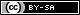# Dev Blog

 ./dev Original theme by orderedlist (CC-BY-SA) Where applicable, all content is licensed under a CC-BY-SA.# awk Cheatsheet

Command Description
echo -e "a b c\nd e f" | awk '{ print }' Print each row
echo -e "a b c\nd e f" | awk '{ print $0 }' Print each row echo -e "a b c\nd e f" | awk '{ print$1, $2,$3 }' Print each element explicitly for each row
echo -e "a\tb\tc\nd\te\tf" | awk -F '\t' '{ print $1,$2, $3 }' Print each element explicitly, using tab as a field separator echo -e "a b c\nd e f" | awk '{ print$(NF-2), $(NF-1),$NF }' Print the last three elements in the row
echo -e "a b c\nd e f" | awk '{ print NR, NF, $0 }' Print row number, number of columns and the row echo -e "a b c\nd e f" | awk '/ e /{ print NR,$0 }' Match regex and then print the row number and the last three fields
echo -e "a b c\nd e f" | awk '$2 == "e" { print NR,$0 }' Test to see if the second field is b and print the row number and row
echo -e "a b c\nd e f" | awk '$2~/e/{ print$0 }' Test if the second field matches regex and print row
echo -e "a b c\nd e f" | awk '{ printf "ok %s\n", $1 }' Use printf to do extra formatting echo -e "1 2 3\n4 5 6" | awk 'BEGIN { print "begin" } { tot = tot +$2 } END { print "end:", tot, tot/NR }' Calculate the running total and print out the sum and average of the second field
ls -la | awk '{ print $1,$2, $3,$4 }' Print out first four columns of ls -la with duplicate separator tokens consolidated into one
echo -e "a\tb\tc\nd\te\tf" | awk 'BEGIN { FS = "\t" } { print NF, $0 }' Change field separator (FS) to tab and print each row and their field count echo -e "a 1\nb 3\nc 7\na 3" | awk '{ print$1,$2; tot[$1]=tot[$1]+$2; count[$1]=count[$1]+1 } END { for (i in count) { print i, tot[i], count[i] } }' Arrays
echo -e "a 1\nb 3\nc 7\na 3" | awk '{ print $1,$2; tot[$1]=tot[$1]+$2; count[$1]=count[\$1]+1 } END { for (i in count) { if (count[i] > 1) print ">1", i, tot[i], count[i] ; else if (count[i] < 2) print "<2", i, tot[i], count[i] } }' Control flow